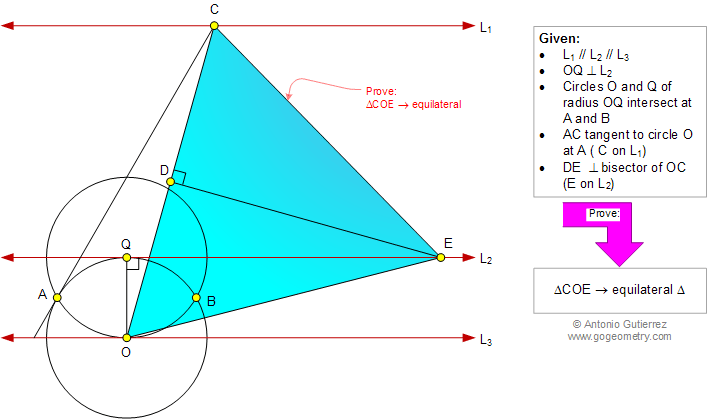# Geometry Problem 972: Equilateral Triangle, Vertices, Three Parallel, Equal Circles, Perpendicular Bisector. Level: School, College, Mathematics Education

< PREVIOUS PROBLEM  |  NEXT PROBLEM >

 Lines L1, L2, and L3 are parallel (Figure below) with OQ perpendicular to L2 (O on L3 and Q on L2). Circles O and Q with radius OQ intersect at A and B. AC is tangent to circle O at A (C on L1). DE is the perpendicular bisector of OC (E on L2). Prove that triangle COE is equilateral.Home | SearchGeometry | Problems | All Problems | Open Problems | Visual Index | 10 Problems | Problems Art Gallery Art | 971-980 | Triangles | Equilateral Triangle | Parallel lines | Perpendicular lines | Circle | Intersecting Circles | Congruence | by Antonio Gutierrez